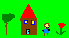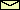# The roots of a polynomial

This is a Java applet which calculates the roots of the polynomial

xn + an-1xn-1 + ... + a1x + a0 = 0.

On the left hand side there are control panels where you can change the order of the polynomial and its coefficients. Just manipulate the scrollbar or enter a number into the number field. The result is show graphically in the complex number plane (x-axis is the real part, y-axis is the imaginary part). On the right-hand side the numerical result is shown. Note that the sign of the real part decides the color code. Below the roots the Hurwitz determinants are shown. Their color code is determined by their sign. They are needed for the Routh-Hurwitz theorem.

Sorry, you need a browser which is capable to execute Java applets. This message also appears for browsers knowing Java but where the Java option is switch off.

Fun with the applet
Here are nice games you can play:
• Try to get all roots onto the left side of the imaginary axis (i.e., the vertical line).
• Try to get all roots on the horizontal line.
The difficulty of these games increases with the order of the polynomial.

# The Routh-Hurwitz theorem

Calculating analytically the roots of a polynomial of order higher than two is very cumbersome and in general impossible if the order is larger than five. But often you do not need the roots of a polynomial explicitely. For example, when you do stability analysis for a fixed point in a (nonlinear) dynamical system you get a characteristic polynomial for the eigenvalues of the Jacobian. To answer the question on stability you don't need the eigenvalues. You only want to know whether all eigenvalues have negative real parts or not. And here comes the neat theorem of Routh and Hurwitz which helps you a lot. It says:

The real part of all roots of the polynomial

xn + an-1xn-1 + ... + a1x + a0 = 0

are negative if and only if all upper-left determinants of the matrix

 an-1

1

an-3

an-2

 0 an-1
 an-5 an-4

an-3

 ... 0 ... 0 ... 0
 : : : a-n+1 a-n+2 a-n+3
 : ... a0

with am=0 for m<0, are positive.Back to my home page

© 1998 Franz-Josef Elmer,Franz-Josef doht Elmer aht unibas doht ch,last modified Thursday, July 16, 1998.